## Before you go, check out these stories!

0ICDAR 2021 Competition: Detecting Tables Using Image Recognition by@pratikkayal

# ICDAR 2021 Competition: Detecting Tables Using Image Recognition### @pratikkayalPratik Kayal

Machine Learning Engineer. Juggling between research and engineering.

To participate go to: https://competitions.codalab.org/competitions/26979

Table recognition is a well-studied problem in document analysis, and many academic and commercial approaches have been developed to recognize tables in several document formats, including plain text, scanned page images, and born-digital, object-based formats such as PDF. There are several works that can convert tables in text-based PDF format into structured representations. However, there is limited work on image-based table content recognition.

The proposed challenge aims at assessing the ability of state-of-the-art methods to recognize scientific tables in LaTeX format. In particular, the problem would be split up into two subtasks:

Subtask I: Table structure reconstruction (S): Reconstructing the structure of a table in the form of LaTeX symbols and code

Subtask II: Table content reconstruction ©: Reconstructing and recognizing the content of a table in the form of LaTeX symbols and code

In this subtask, you are given an image of a table and its corresponding LaTeX code. You need to construct the LaTeX structural tokens that define the table in LaTeX.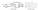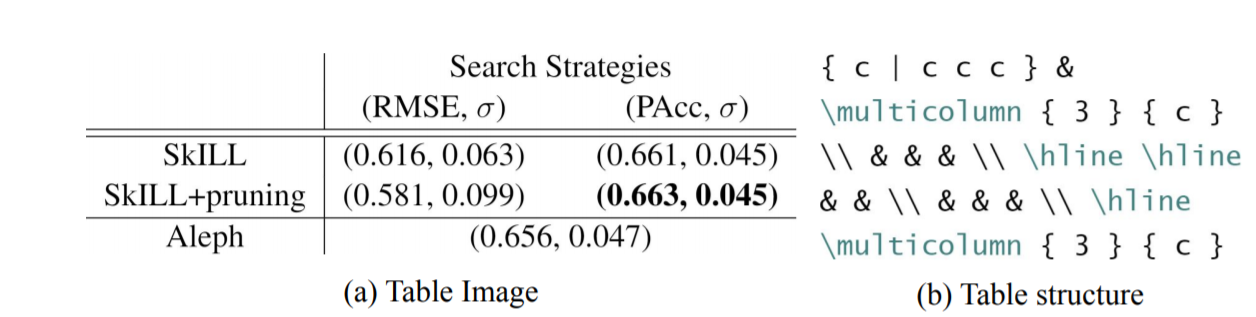In this subtask, you are given an image of a table and its corresponding LaTeX code. You need to construct the LaTeX content tokens that belong to the table in LaTeX.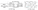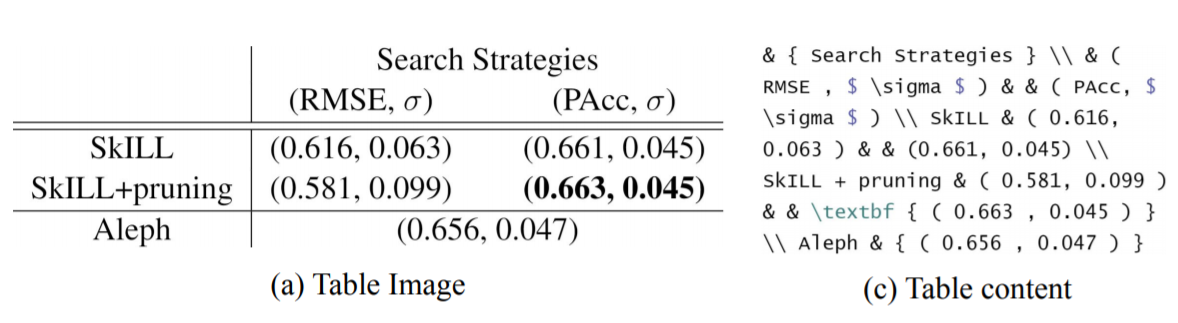Q1. What is the size of the dataset with specific numbers for each task (training set — test — validation set)?

A1. Size of the dataset for both the subtasks is given as follows: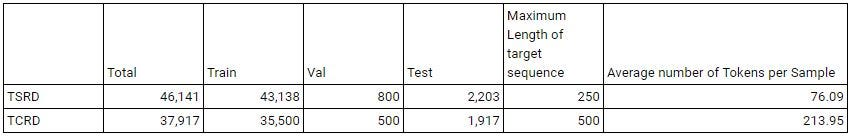We abbreviate Table structure reconstruction task dataset as TSRD and Table content reconstruction task dataset as TCRD.

For the TSR dataset, we take tables having less than 250 tokens and for TCR dataset we take tables having less than 500 tokens.

Q2. Will the code of the competitors be available for the research community (reproducibility of the results)?

A2. It would be mandatory for participants to make their code available for reproducibility. The dataset provided for this task would be licensed under CC BY-NC-SA 4.0 international license, and the evaluation script would be provided under MIT License.

Q3. Will there be an award for all the proposed Tasks?

A3. We would be awarding both the proposed subtasks:

Q4. What are some Examples for the two tasks?

A4. Examples:

Table Structure Reconstruction: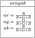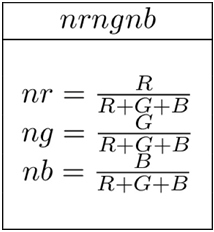{ | c c | } \\hline \\multicolumn { 2 } { | c | } CELL \\\\ \\hline \\multicolumn { 2 } { | c | } CELL \\\\ \\multicolumn { 2 } { | c | } CELL \\\\ \\multicolumn { 2 } { | c | } CELL \\\\ \\multicolumn { 2 } { | c | } CELL \\\\ \\multicolumn { 2 } { | c | } CELL \\\\ \\hline

Table Content Reconstruction: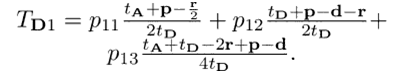$T _ { \mathbf { D } 1 } = p _ { 1 1 ¦ } \frac { t _ { \mathbf { A } } + \mathbf { p } — \frac { \mathbf { r } } { 2 } } { 2 t ¦ _ { \mathbf { D } } } + p _ { 1 2 ¦ } \frac { t _ { \mathbf { D } } + \mathbf { p — d — r } } { 2 t ¦ _ { \mathbf { D } } } +$ \\ $p _ { 1 3 ¦ } \frac { t _ { \mathbf { A } } + t _ { \mathbf { D } } — 2 \mathbf { r + p — d } } { 4 t ¦ _ { \mathbf { D } } } .$

# Timeline:

Registration Period: 15th Oct 2020 to 28th Feb 2021Release of training and validation set: 20th Oct 2020Release of test set: 01st Mar 2021Submission Deadline: 31st Mar 2021Post-Evaluation Phase Starts: 01st Apr 2021

# Evaluation

For both the subtasks, the participants would be required to submit the prediction files as per the submission format.

The tasks would be scored by Exact Match Accuracy and Exact Match Accuracy @ 95% similarity as common evaluation metrics.

Row Prediction Accuracy and Column Prediction Accuracy for Table structure reconstruction taskAlpha-Numeric characters Prediction Accuracy, LaTeX Token Accuracy, LaTex Symbol Accuracy, and Non-LaTeX Symbols Prediction Accuracy for Table content reconstruction task

The description of each metric is as follows:

• Exact Match Accuracy: Fraction of predictions which match exactly with the ground truth
• Exact Match Accuracy @ 95% similarity: Fraction of predictions with at least 95% similarity between ground truth
• Row Prediction Accuracy: Fraction of predictions with a count of rows equal to the count of rows in the ground truth
• Column Prediction Accuracy: Fraction of predictions with a count of cell alignment (‘c’, ‘r’, ‘l’) tokens equal to the count of cell alignment tokens in the ground truth
• Alpha-Numeric Characters Prediction Accuracy: Fraction of predictions which has the same alphanumeric characters as in the ground truth
• LaTeX Token Accuracy: Fraction of predictions which has the same LaTeX tokens as in the ground truth
• LaTeX Symbol Accuracy: Fraction of predictions which has the same LaTeX Symbols as in the ground truth
• Non-LaTeX Symbol Prediction Accuracy: Fraction of predictions which has the same Non-LaTeX Symbols as in the ground truth

Example: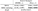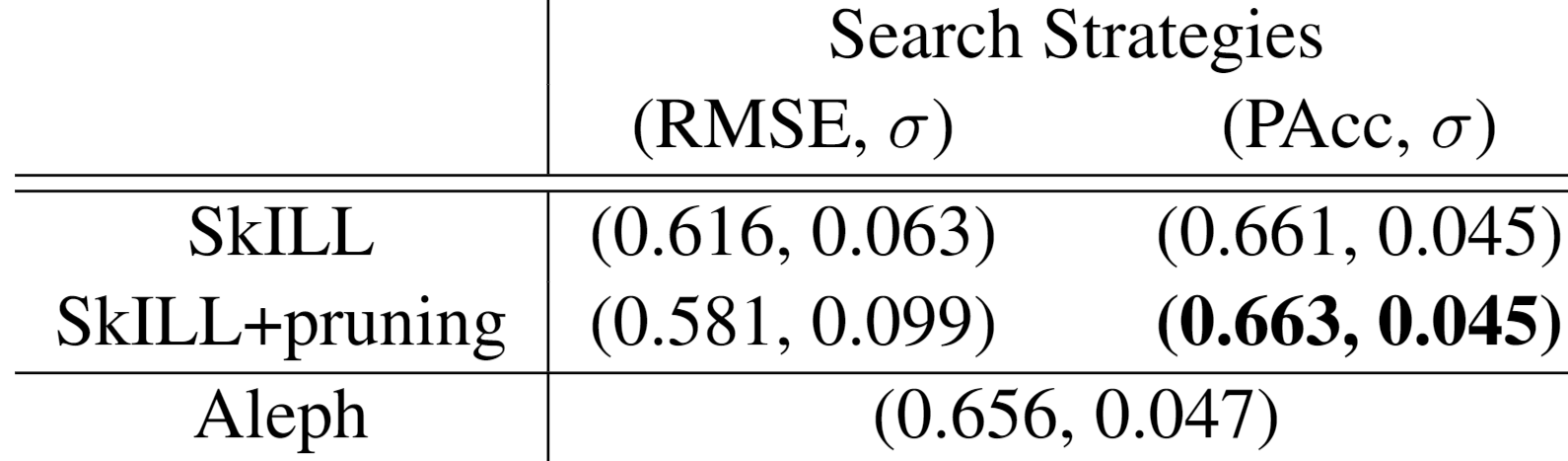For the given image, to calculate Exact Match Accuracy @ 95% similarity between the ground truth target sequence and predicted target sequence, we use the Longest Common Subsequence algorithm to find the similarity percentage and set the similarity percentage minimum threshold to 95%.

The ground truth target sequence (G) for Table structure recognition task is { c | c c c } & \milticolumn { 3 } { c } \\ & & & \\ \hline \hline & & \\ & & & \\ \hline \multicolumn { 3 } { c } (No. of tokens = 37)

and the predicted target sequence (P) is { c | c c } & \milticolumn { 2 } { c } \\ & & & \\ \hline \hline & & \\ & & & \\ \hline \multicolumn { 3 } { c } (No. of tokens = 36)

The longest common subsequence between G and P is } { c } \\ & & & \\ \hline \hline & & \\ & & & \\ \hline \multicolumn { 3 } { c }.

Thus, the percentage similarity calculated is 70.27% (26/.37).